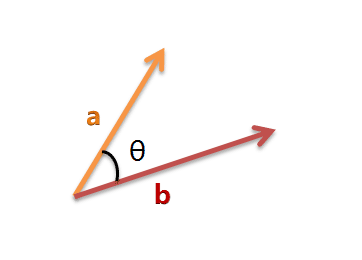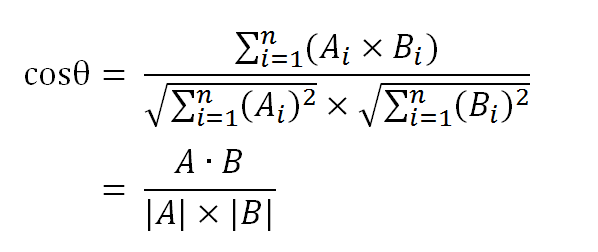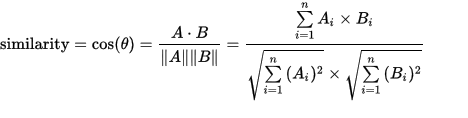# Word2Vec - 余弦相似性数学原理（1/3）A： 我喜欢足球，也喜欢篮球。

B： 我喜欢足球，不喜欢篮球。

## 对句子进行分词，并统计词频

A：我/ 喜欢/ 足球/ ,/ 也/ 喜欢/ 篮球 /。

B：我/ 喜欢/ 足球/ ,/ 不/ 喜欢/ 篮球/ 。

A：我：1，喜欢：2，足球：1，篮球：1，也：1，不：0

B：我：1，喜欢：2，足球：1，篮球：1，也：1，不：1

A：[1, 2, 1, 1, 0]

B：[1, 2, 1, 1, 1]

## 计算相似性

### 过程

(1）使用TF-IDF算法，找出两篇文章的关键词；

(2）每篇文章各取出若干个关键词（比如20个），合并成一个集合，计算每篇文章对于这个集合中的词的词频（为了避免文章长度的差异，可以使用相对词频）；

(3）生成两篇文章各自的词频向量；

(4）计算两个向量的余弦相似度，值越大就表示越相似。# -*- coding: utf8 -*-
import math
import jieba.analyse
article_a = '我喜欢中国，也喜欢美国。'
article_b = '我喜欢足球，不喜欢篮球。'

def cut_word(article):
# 这里使用了TF-IDF算法，所以分词结果会有些不同->https://github.com/fxsjy/jieba#3-关键词提取
res = jieba.analyse.extract_tags(
sentence=article, topK=20, withWeight=True)
return res

def tf_idf(res1=None, res2=None):
# 向量，可以使用list表示
vector_1 = []
vector_2 = []
# 词频，可以使用dict表示
tf_1 = {i: i for i in res1}
tf_2 = {i: i for i in res2}
res = set(list(tf_1.keys()) + list(tf_2.keys()))

# 填充词频向量
for word in res:
if word in tf_1:
vector_1.append(tf_1[word])
else:
vector_1.append(0)
if word in tf_2:
vector_2.append(tf_2[word])
else:
vector_2.append(0)

return vector_1, vector_2

def numerator(vector1, vector2):
#分子
return sum(a * b for a, b in zip(vector1, vector2))

def denominator(vector):
#分母
return math.sqrt(sum(a * b for a,b in zip(vector, vector)))

def run(vector1, vector2):
return numerator(vector1,vector2) / (denominator(vector1) * denominator(vector2))

vectors =  tf_idf(res1=cut_word(article=article_a), res2=cut_word(article=article_b))
# 相似度
similarity = run(vector1=vectors, vector2=vectors)
# 使用arccos计算弧度

# 0.2157074518785444 1.353380046633586


## 参考

TF-IDF与余弦相似性的应用（二）：找出相似文章

Chatopera博客 聊天机器人 机器学习 智能客服01-0854
11-102124
10-07437
01-1530
01-0886
01-0799
01-0275
01-0273
12-29603
12-293839
12-2656
12-25237
12-2563
12-01154
11-30264
11-12263
11-07562
11-03371
11-03159
10-20436